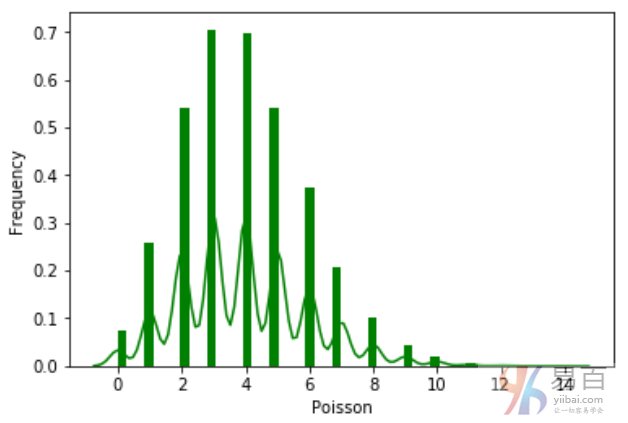# (39)Python泊松分布

``````from scipy.stats import poisson
import seaborn as sb

data_binom = poisson.rvs(mu=4, size=10000)
ax = sb.distplot(data_binom,
kde=True,
color='green',
hist_kws={"linewidth": 25,'alpha':1})
ax.set(xlabel='Poisson', ylabel='Frequency')
```Python```关注右侧公众号，随时随地查看教程
Python数据分析教程目录